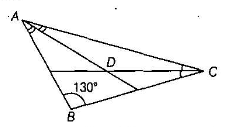Home/Class 9/Maths/

If one of the angles of a triangle is $$130°$$, then find the angle between the bisectors of the other two angles.Speed
00:00
02:45## QuestionMathsClass 9

If one of the angles of a triangle is $$130°$$, then find the angle between the bisectors of the other two angles.We are given that $$\angle\;B=130°$$
Now we know the angle sum property of triangles that the sum of interior angles of a triangle is equal to $$180°$$
So $$\angle\;A+\angle\;B+\angle\;C=180°$$
Putting the value of $$\angle\;B$$ in the above equation, we get,
$$\angle\;A+130°+\angle\;C=180°$$
$$\angle\;A+\angle\;C=180°-130°$$
$$\angle\;A+\angle\;C=50°$$
Now dividing by 2
$$\frac{1}{2}\angle\;A+\frac{1}{2}\angle\;C=25° ...(1)$$
Also, we can apply the sum of angles property in $$∆ADC$$.
Thus, we have,
$$\frac{1}{2}\angle\;A+\frac{1}{2}\angle\;C+\angle\;D=180° ...(2)$$
Now, substituting the value of (1) in equation (2), we get,
$$25°+\angle\;D=180°$$
$$\angle\;D=180°-25°$$
$$\angle\;D=155°$$
Therefore, the angle between the bisectors of the other two angles is $$155°$$.